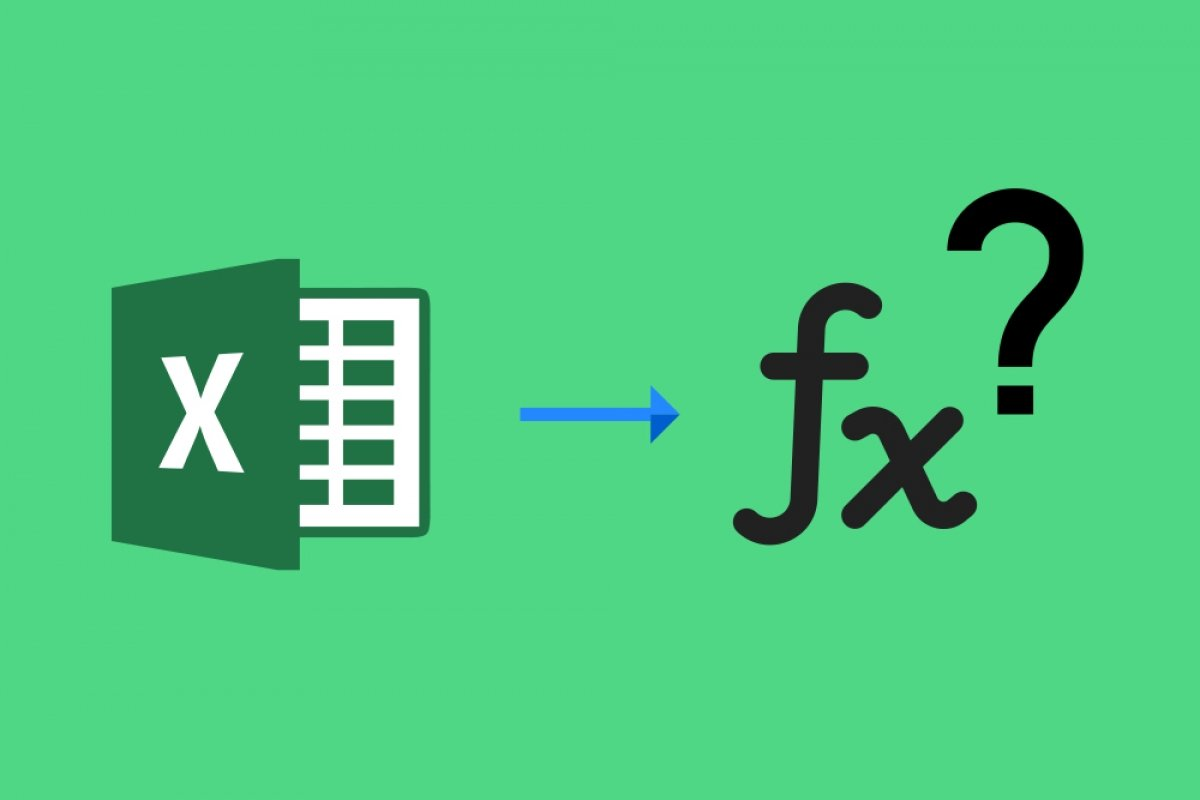An Excel function is a predefined mathematical formula that performs automatic calculations. To perform them, it uses specific values and follows a particular order. The user can define the functions and their main value is that they save time, since it is not necessary to perform all the calculations each time they are needed.

The functions obey a very specific syntax that must be followed, otherwise, we will get an error. The steps to follow are:

1. Insert the "=" sign.
2. Select a function.
3. Enter the data to be used to operate (also called formula arguments). They obey specific cells.

As an example, suppose we want to add the values of two cells. Let's say we have two cells with two values, A1 and B1: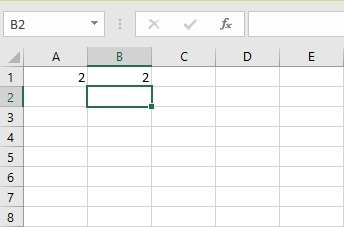Values in Excel columns

We want to add these values. How will we do it? Well, following the syntax rule we showed above, we should write =SUM(A1:B1) in the cell where we want to see the result: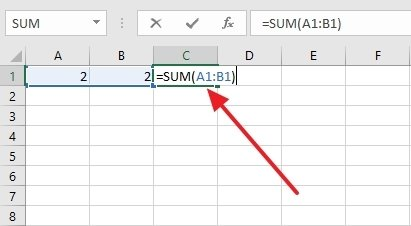Sum of the values of the columns

To separate the arguments, you must use a colon and commas. Commas are used to separate sets of arguments: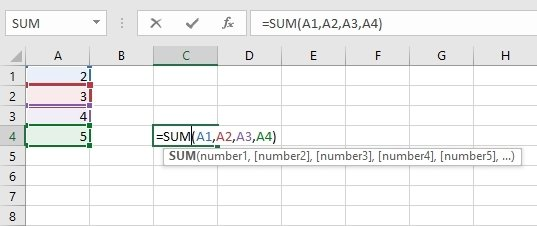Sum of the values from A1 to A4

In this example, we add the arguments from cell A1 to cell A4.

The colon will execute the operation between cells or ranges of cells: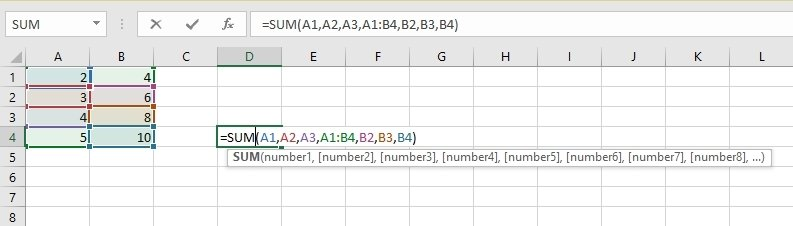Sum of the ranges from A1 to A4 and B1 to B4

In this other example, we add the arguments that go from column A1 to A4, and those that go from B1 to B4.

The sum is only a minimal representation of all the functions available in Excel. There are more than 200 functions available that are grouped into different categories: mathematics and trigonometry, financial, statistics, among others. If you need to do an operation in Excel, surely there is a function for it.